# Quiz 13: Real-Options Analysis

A call option gives its owner the right, but not the compulsion, to buy a fixed number of shares at the strike price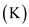on the maturity date. Given Stock price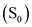= \$120, Strike price= \$150, risk free interest rate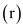=6 %, volatility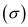= 45% and Time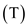=0.5 years and it is required to find out the price of a European call option. The Black-Scholes formula for the price of the call is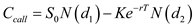Where,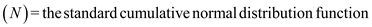Substitute the values in the above formula and can be seen as follows: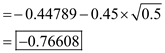Now, after getting the values of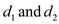, it is required to get the normal distributive value from the appendix C of the book and the values will be,The call option value will be calculated as done below:The price for the call will be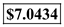A call option gives its owner the right, but not the compulsion, to buy a fixed number of shares at the strike price on the maturity date. Given the value of project to be \$100 million and rate of interest of 6%. (a) It is needed to calculate the underlying asset lattice which can be calculated with the appropriate formula in the excel as seen below: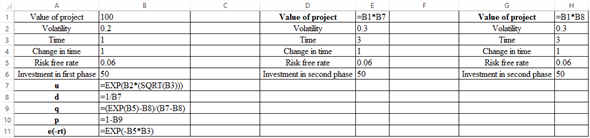The corresponding values are: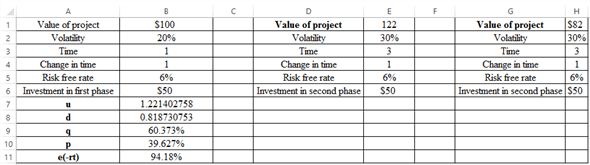Hence, it can be seen that the value of the project changes over time. It can be better understood by the diagram given below: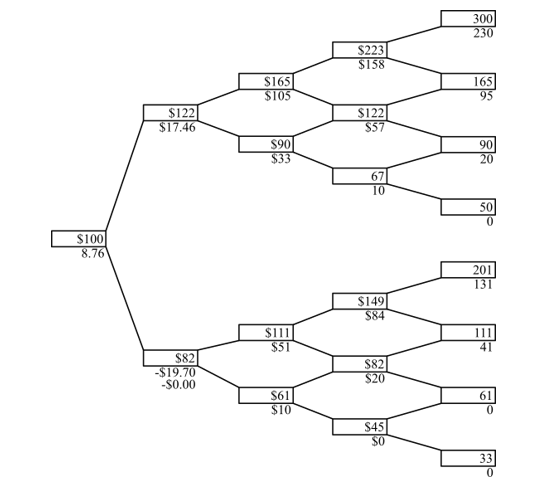(b) The option value can be better calculated from the diagram given below: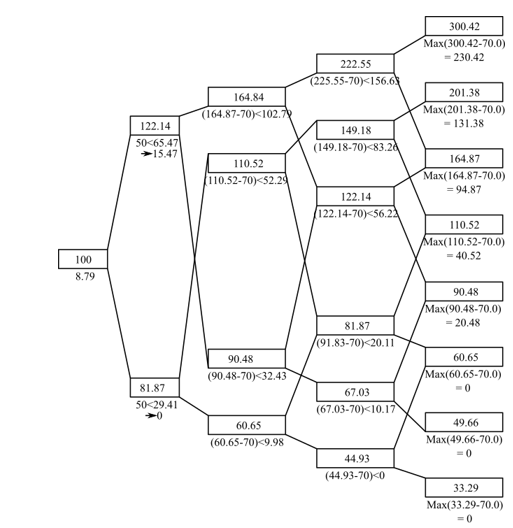Hence, the option value is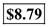.
A put option is a security or collateral that you buy when you consider the price of a stock is going to go down. It is the right to sell the shares of a stock at a specified price and that "specified price" is recognized as the strike price. Given Stock price= \$68.50, Strike price= \$70, risk free interest rate=5 %, volatility= 60% and Time=0.16 years and it is required to find out the price of a European put option. The Black-Scholes formula for the put option is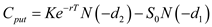Where,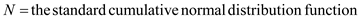Substitute the values in the above formula and can be seen as follows: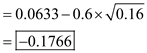Now, after getting the values of, it is required to get the normal distributive value from the appendix C of the book and the values will be,The put option value will be calculated as done below:The price for the call will be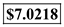.